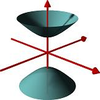#### You may also like### A Close Match

Can you massage the parameters of these curves to make them match as closely as possible?### Prime Counter

A short challenge concerning prime numbers.### The Right Volume

Can you rotate a curve to make a volume of 1?

# Trig Trig Trig

##### Age 16 to 18 ShortChallenge Level

Consider the function $f(x)=\cos(\sin(\cos(x)))$, with $x$ measured in radians.

What turning points can you find?

What are the maximum and minimum values of the function?

Did you know ... ?

This function is bounded, continuous and differentiable at all points. Mathematicians often use knowledge of conditions such as these to deduce lots of information about the properties of functions without the need for extensive calculation. In first year undergraduate analysis courses theorems are rigorously stated and proved which support intuitive statements such as 'between any two maxima a minimum must be found if the function is finite, continuous and differentiable'.